#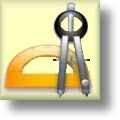Geometry Worksheets

Here is a graphic preview for all of the Quadrilaterals and Polygons Worksheets Sections. You can select different variables to customize these Quadrilaterals and Polygons Worksheets for your needs. The Quadrilaterals and Polygons Worksheets are randomly created and will never repeat so you have an endless supply of quality Quadrilaterals and Polygons Worksheets to use in the classroom or at home. We have area & perimeter worksheets, identification of quadrilaters and polygons worksheets, and angle of quadrilaterals and polygons worksheets. Our Quadrilaterals and Polygons Worksheets are free to download, easy to use, and very flexible.

Click the image to be taken to that Quadrilaterals and Polygons Worksheets.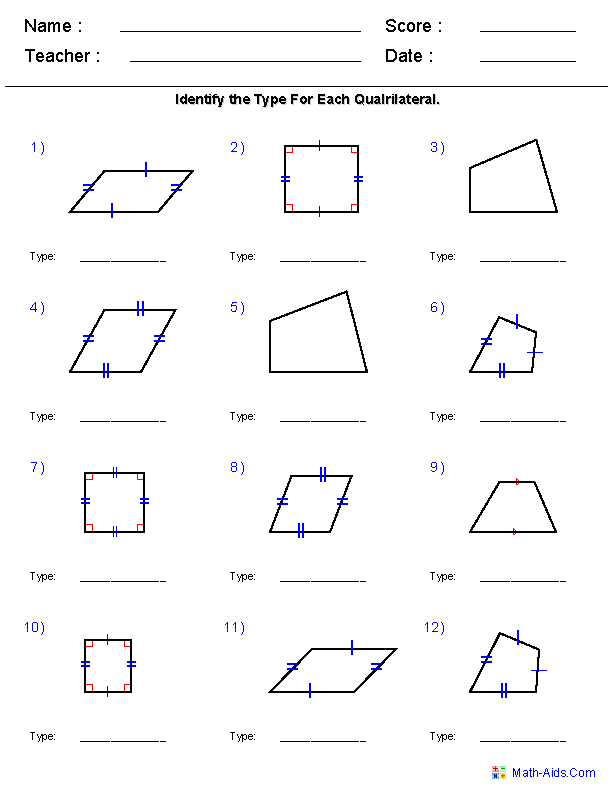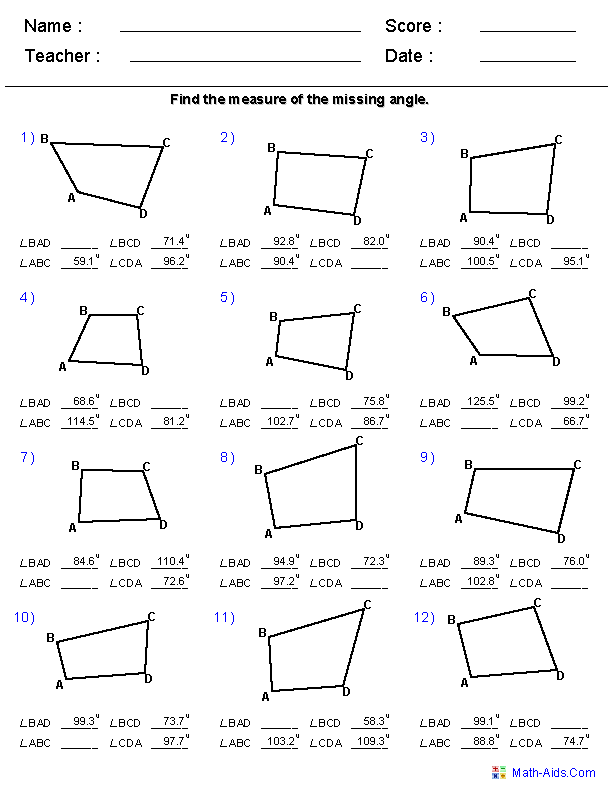##### Area and Perimeter ofQuadrilaterals Worksheets##### Identify Regular PolygonsWorksheets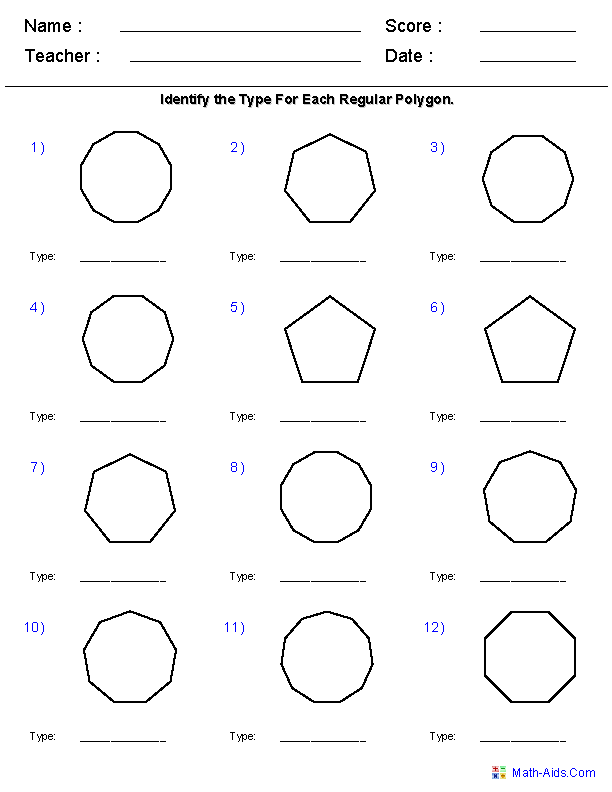##### Angles of Regular PolygonsWorksheets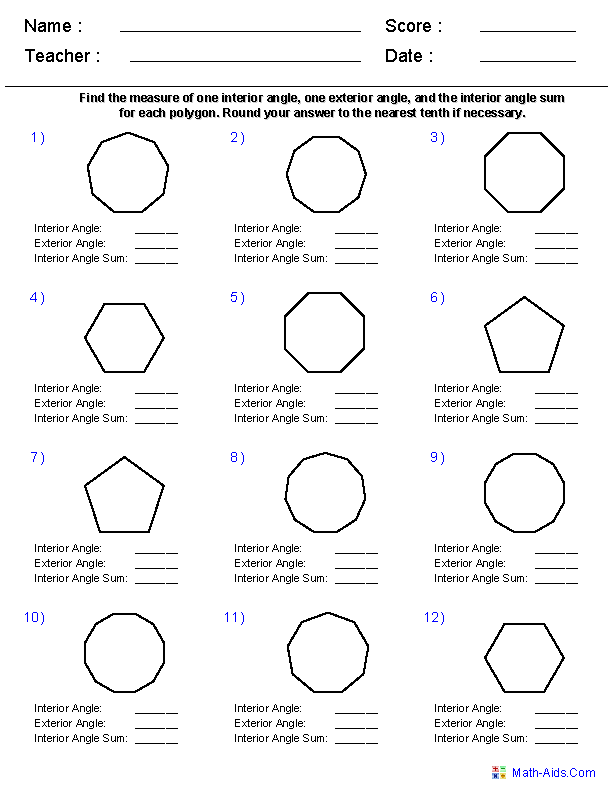##### Area and Perimeter ofRegular Polygons Worksheets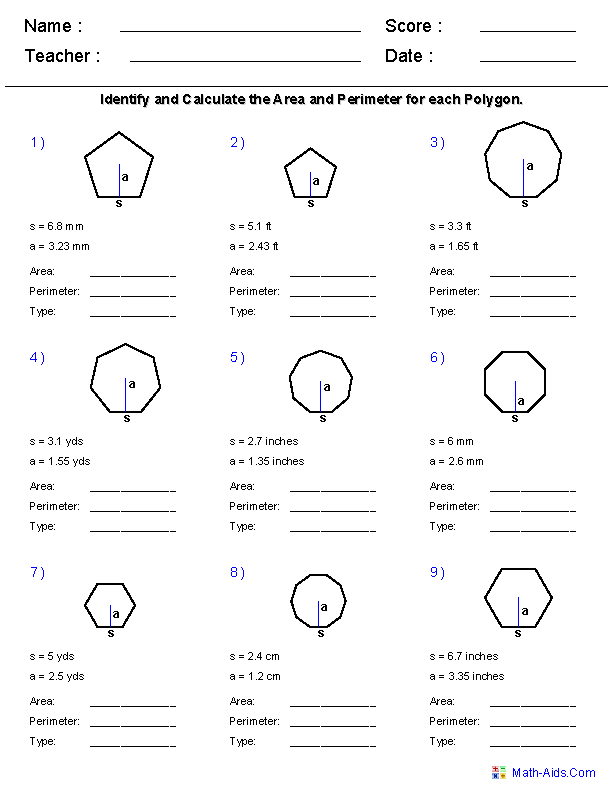##### Area and Perimeter UsingAll Polygons Worksheets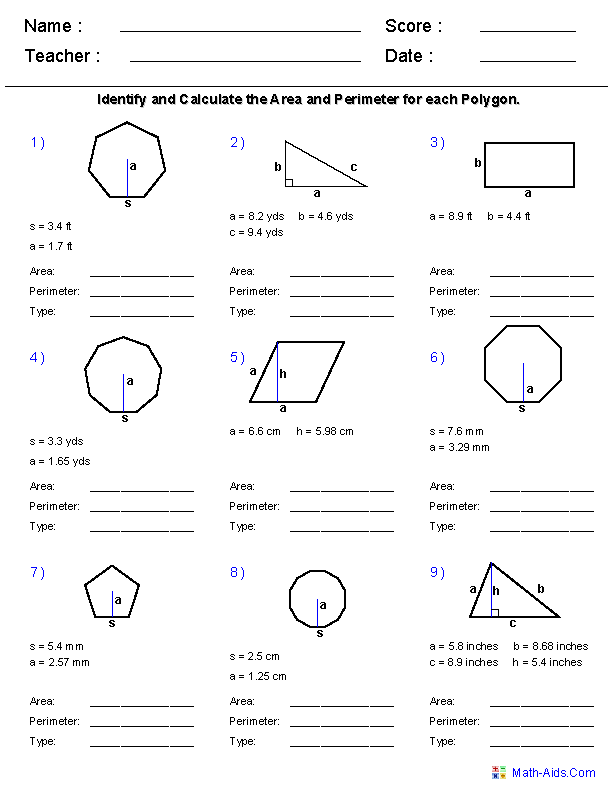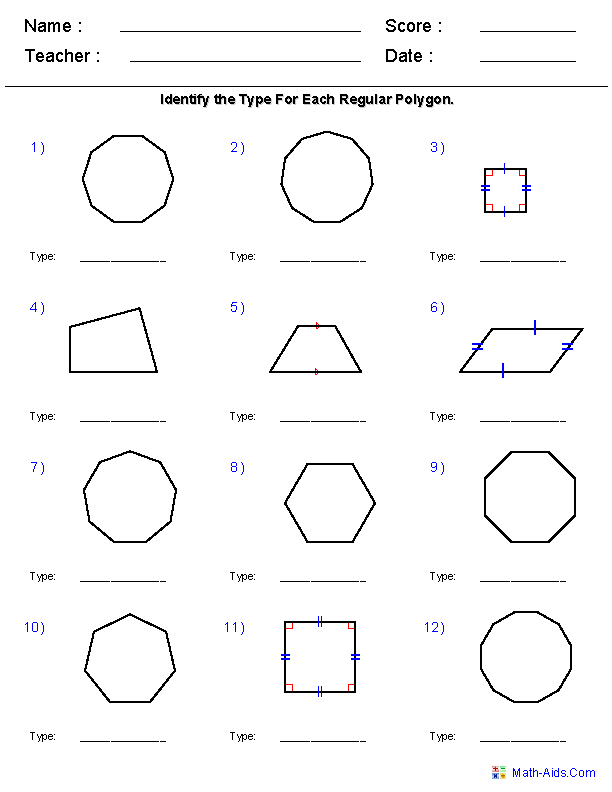##### Properties of ParallelogramsWorksheets##### Properties of TrapezoidsWorksheetsRecommended Videos

## Detailed Description for All Quadrilaterals and Polygons Worksheets

These Quadrilaterals and Polygons Worksheets will produce twelve problems for identifying different types of quadrilaterals. You may select squares, rectangles, parallelograms, rhombuses, trapezoids, kites, and quadrilaterals. These worksheets are a great resources for the 5th, 6th Grade, 7th Grade, and 8th Grade.

Interior Angles of a Quadrilateral Worksheets
These Quadrilaterals and Polygons Worksheets will produce twelve problems for finding the interior angles of randomly generated quadrilaterals. You may select the number of decimals for the angles. This worksheet is a great resources for the 5th, 6th Grade, 7th Grade, and 8th Grade.

Area and Perimeter of Quadrilaterals Worksheets
These Quadrilaterals and Polygons Worksheets will produce nine problems for solving the area and perimeter for squares, rectangles, parallelograms, rhombuses, and trapezoids. These worksheet are a great resources for the 5th, 6th Grade, 7th Grade, and 8th Grade.

Identify Regular Polygons Worksheets
These Quadrilaterals and Polygons Worksheets will produce twelve problems for identifying different types of regular polygons. You may select pentagons, hexagons, heptagons, octagons, nonagons, decagons, hendecagons, and dodecagons. These worksheets are a great resources for the 5th, 6th Grade, 7th Grade, and 8th Grade.

Angles of Regular Polygons Worksheets
These Quadrilaterals and Polygons Worksheets will produce twelve problems for solving the interior and exterior angles of different regular polygons. You may select pentagons, hexagons, heptagons, octagons, nonagons, decagons, hendecagons, and dodecagons. These worksheet are a great resources for the 5th, 6th Grade, 7th Grade, and 8th Grade.

Area and Perimeter of Regular Polygons Worksheets
These Quadrilaterals and Polygons Worksheets will produce nine problems for solving the area and perimeter for pentagons, hexagons, heptagons, octagons, nonagons, decagons, hendecagons, and dodecagons. These worksheet are a great resources for the 5th, 6th Grade, 7th Grade, and 8th Grade.

Area and Perimeter Using All Polygons Worksheets
These Quadrilaterals and Polygons Worksheets will produce nine problems for solving the area and perimeter for right triangles, common triangles, equilateral triangles, isosceles triangles, squares, rectangles, parallelograms, rhombuses, trapezoids, pentagons, hexagons, heptagons, octagons, nonagons, decagons, hendecagons, and dodecagons. These worksheet are a great resources for the 5th, 6th Grade, 7th Grade, and 8th Grade.

These Quadrilaterals and Polygons Worksheets will produce twelve problems for solving the Area and Perimeter of different types of polygons. You may select squares, rectangles, parallelograms, rhombuses, trapezoids, kites, quadrilaterals, pentagons, hexagons, heptagons, octagons, nonagons, decagons, hendecagons, and dodecagons. These worksheets are a great resources for the 5th, 6th Grade, 7th Grade, and 8th Grade.

Properties of Parallelograms Worksheets
These Quadrilaterals and Polygons Worksheets will produce twelve problems for finding the interior angles and lengths of sides for different parallelograms. You may select between whole and decimal numbers, as well as whether the properties will have algebraic expressions to solve. These worksheets are a great resources for the 5th, 6th Grade, 7th Grade, and 8th Grade.

Properties of Trapezoids Worksheets
These Quadrilaterals and Polygons Worksheets will produce twelve problems for finding the interior angles and lengths of sides for different trapezoids. You may select between whole and decimal numbers, as well as whether the properties will have algebraic expressions to solve. These worksheets are a great resources for the 5th, 6th Grade, 7th Grade, and 8th Grade.